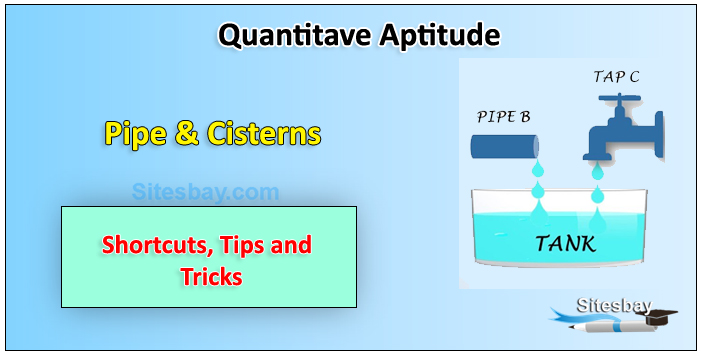# Pipes and Cisterns Problems

## Pipes and Cisterns Problems

In every Written test at leat 1 or 2 questions are asked from this topic. Here we discuss this topic using simple tips and shortcut methods.Inlet: A pipe connected with a tank or reservoir for filling is called as inlet

Outlet: A pipe connected with a tank and used for empties it is called outlet.

Rule: If a pipe can fill a tank in x hours, then the part filled in 1 hour = 1 / x

Rule: If a pipe can fill a tank in x hours and another pipe can empty the full tank in y hours, then the net part filled in 1 hour, when both the pipes are opened.

## Solution

```(1/X-1/Y)
```

Time taken to fill the tank, when both the pipes are opened

## Solution

```(XY/Y-X)
```

Rule: If a pipe can fill a tank in x hours and another fill the same tank in y hours, then the net part filled in 1 hr, when both pipes are opened.

## Solution

```(1/X+1/Y)

So time to fill the tank will be

(XY/Y+X)
```

Rule: If a pipe fills a tank in x hrs and another fills the same tank in y hrs, but a third empties the full tank in z hrs and all of them are opened together, the net part filled in 1 hr.

## Solution

```(1/X+1/Y-1/Z)

So time taken to fill the tank

(XYZ/YZ+XZ-Y)
```

A tank is filled by three pipes A, B and C. The pipe C is twice as fast as B and B is twice as fast as A. How much time will pipe A alone take to fill the tank ?

## Solution

```Solution : Let Pipe A take x hours to fill the tank , then pipes B will take x / 2 hrs and pipe C will take x / 4 hours respectively.

Then part to be filled by all three pipes in 1 hrs will be :

1/X+2/X+4/X = 1/5

X=35 hrs
```

Example: Two pipes A and B can fill a tank in 15 minutes and 20 minutes respectively. Both the pipes are opened together but after 4 minutes, Pipe A is turned off. What is the total time required to fill the tank ?

Solution: Part of tank filled by A + B in 1 minute = ( 1 / 15 + 1 / 20 ) So tank filled by A + B in 4 minute = 4 ( 1 / 15 + 1 / 20 ) = 7 / 15 Part remaning = 1 - ( 7 / 15 ) = 8 / 15 1 / 20 part is filled by B in 1 minute

So, 8 / 15 part will be filled in = ( 20 / 1 )* ( 8 / 15 ) = 32 / 3 = 10 minutes 40 sec.